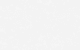## Modulus of Elasticity of Concrete Calculator

Calculate the modulus of elasticity of concrete in accordance to different design codes.

Most design codes have different equations to compute the elastic modulus of concrete. Brief Description Modulus of elasticity is one of the most important properties of concrete, or any material for that matter, because it represents the capacity of the material to resist deformation under applied load. This property is the basis of our understanding … Read more about Modulus of Elasticity of Concrete Calculator